# Quotient

Go back to  'PEMDAS'

 1 Introduction to Division 2 What is Division? 3 Terms Related to Division 4 What are Quotients 5 Long Division Method 6 Tips and Tricks 7 Quotient Calculator 8 Solved Examples on Division 9 Practice Questions on Division 10 Challenging Questions on Division 11 Maths Olympiad Sample Papers 12 Frequently Asked Questions (FAQs)

## Introduction to Division

Division is one of the four basic mathematical operations, the other three being Addition, Subtraction and Multiplication.

In simple words, division can be defined as the splitting of a large group into equal smaller groups.

Division can be introduced by considering objects from our daily life like slices of pizza or a bar of chocolate.

Look at the images below. In the first image, we see a pizza is divided into 4 slices.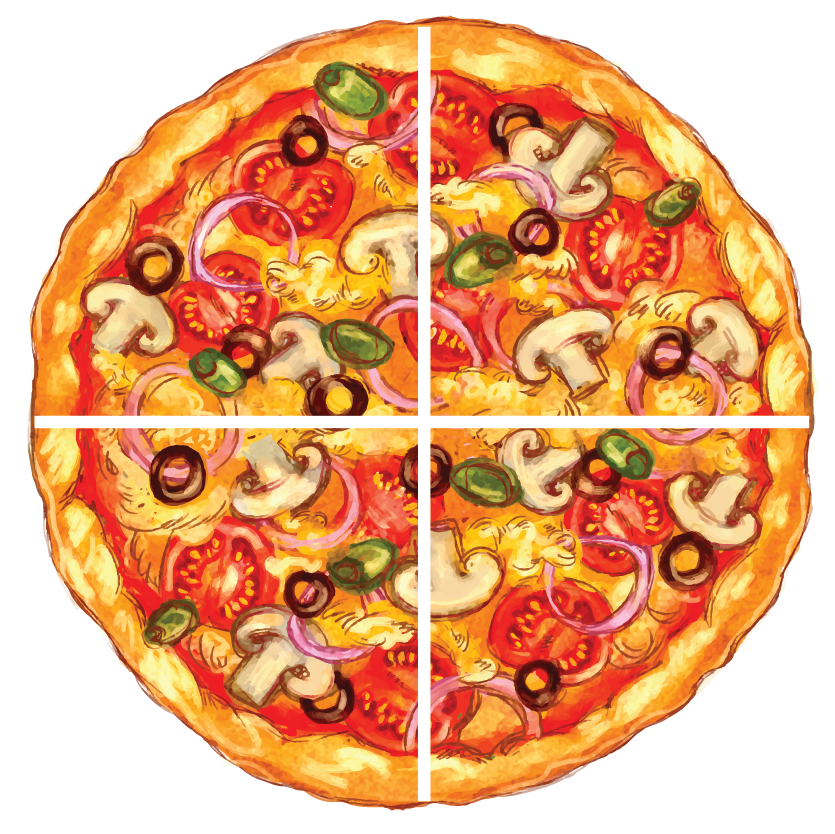In the second image, a chocolate bar with 12 pieces is divided into mini chocolate bars with 6 pieces each.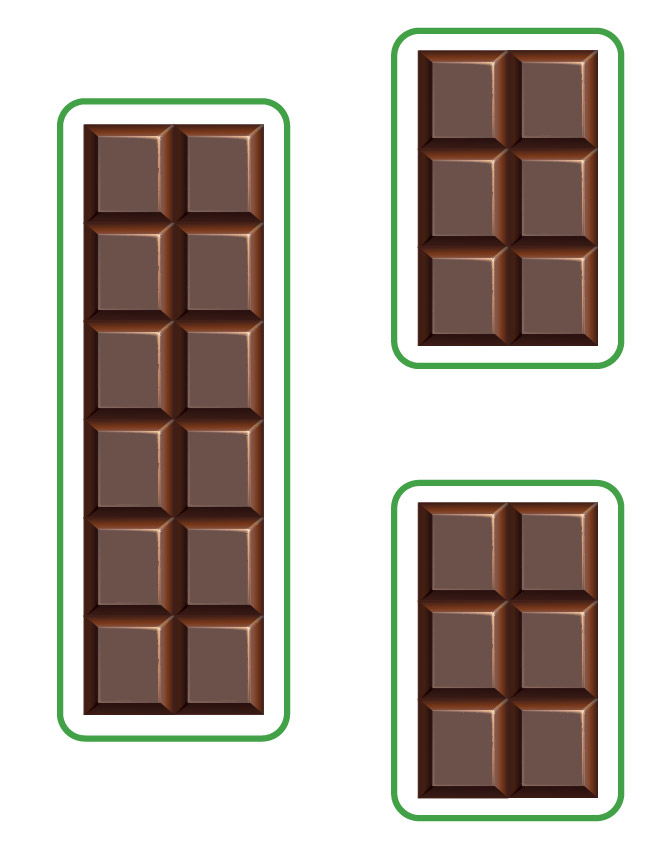## What is Division?

Division is the process of repetitive subtraction.

Division is denoted by a mathematical symbol which consists of a short horizontal line with a dot each above and below the line.Let us explore division with few examples.

Example 1:

The example shows division as a method of grouping objects equally in groups.

The box below contains 16 balls.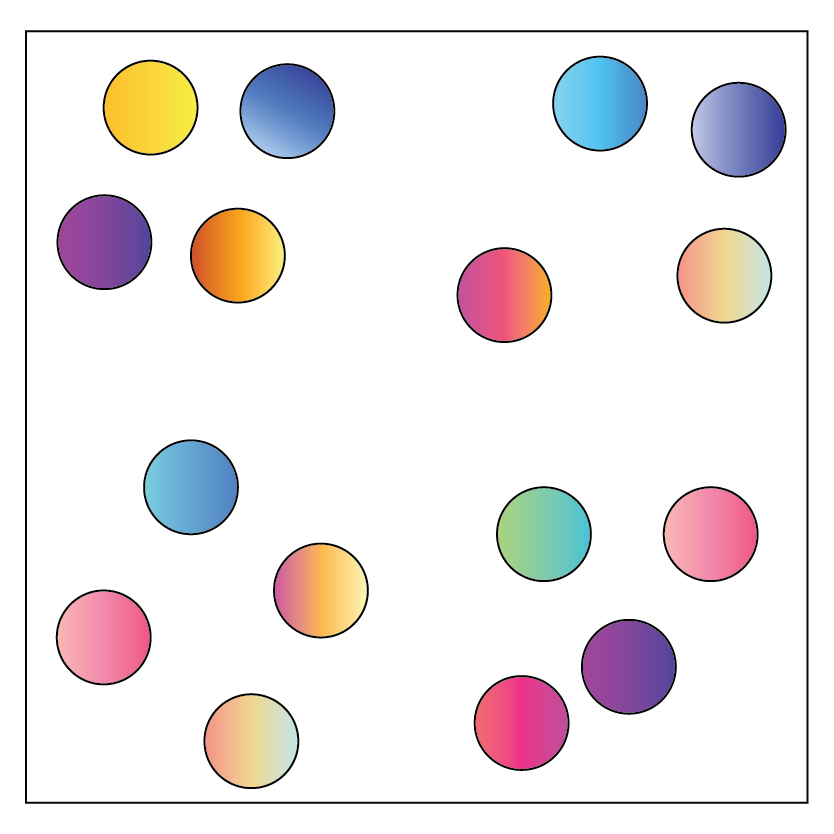Let us divide them into 4 equal groups.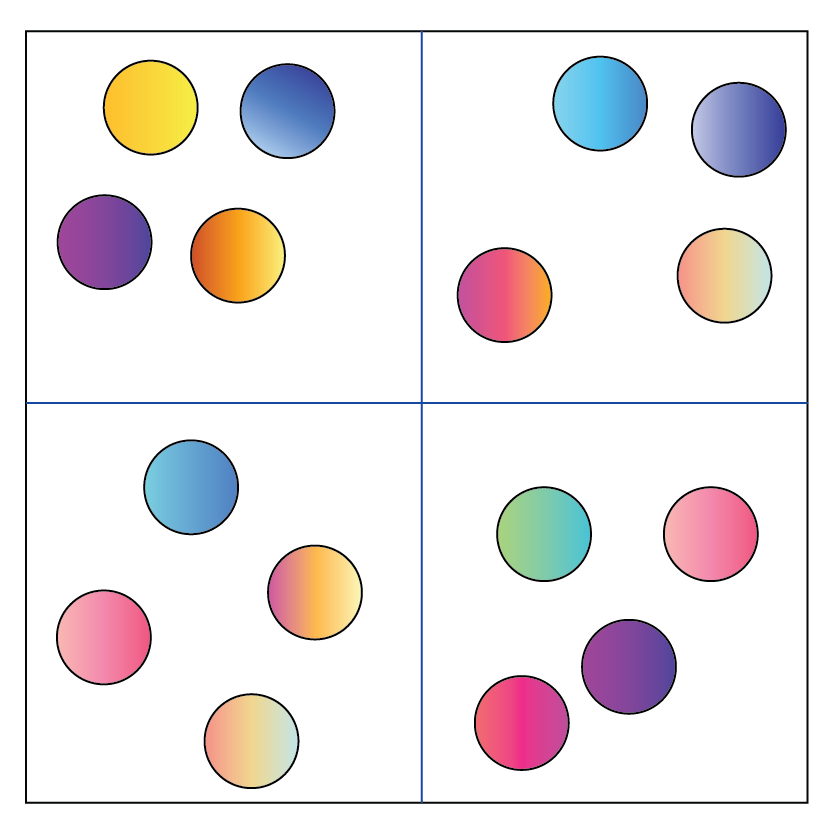We can see in the above figure that there are 4 balls placed in each group.

The division statement for the picture above can be written as

$16\div4=4$

Example 2:

The following image shows 14 fish from the larger bowl being equally distributed into two smaller bowls.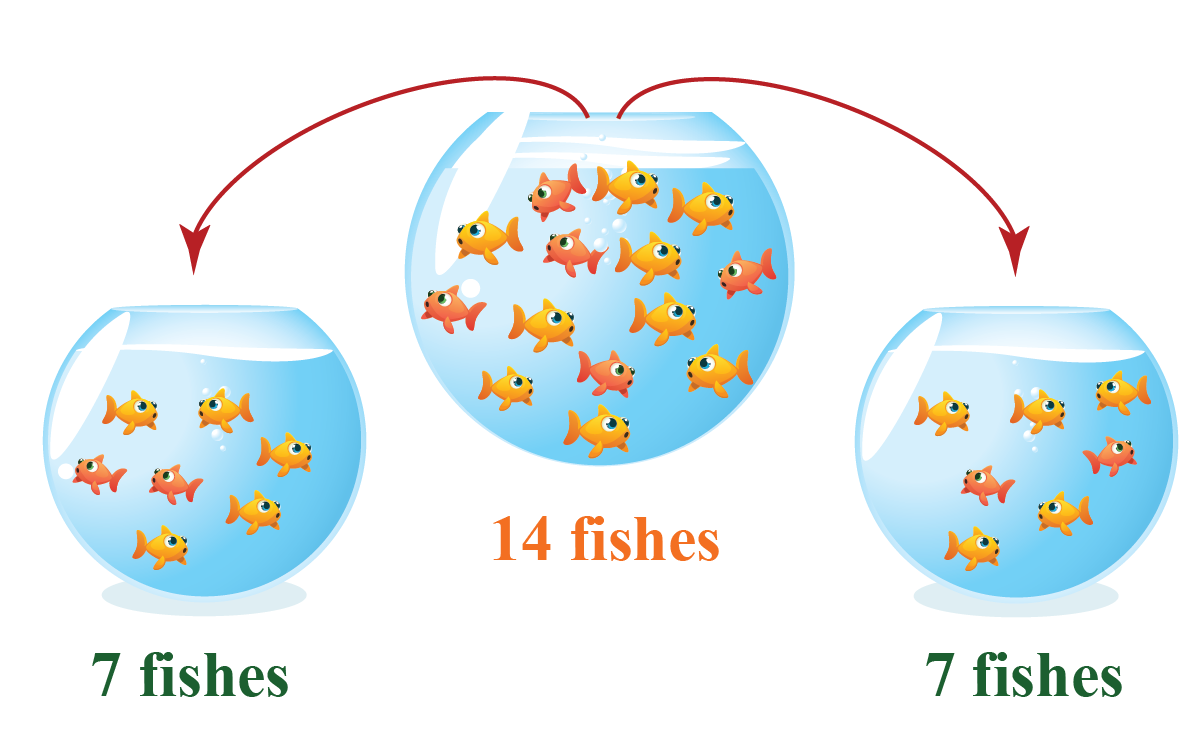Division as repeated subtraction can be seen here. As you can see, there are 14 fish in the larger bowl.

We have to divide them equally into the two smaller bowls.

By taking every fish (subtracting) from the large bowl and placing them in the small bowls alternately, we get 7 fish in each small bowl.

The division statement for the picture above can be written as

$14 \div 2=7$

Example 3:

Let us now divide this bar of chocolate having 12 pieces into 3 equal parts.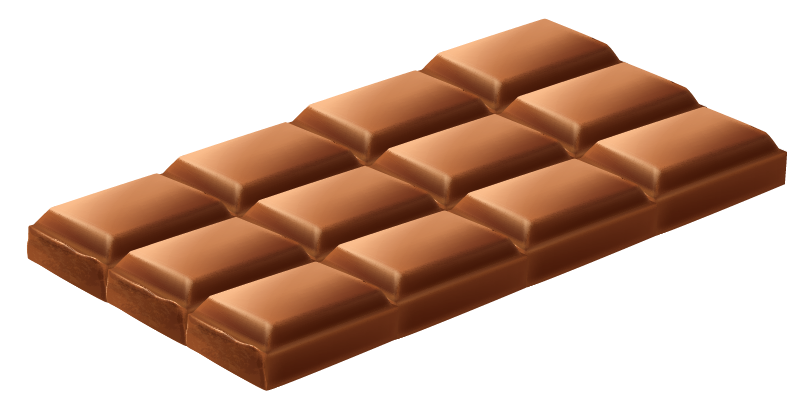By dividing the bar of chocolate, we get 4 pieces in each part.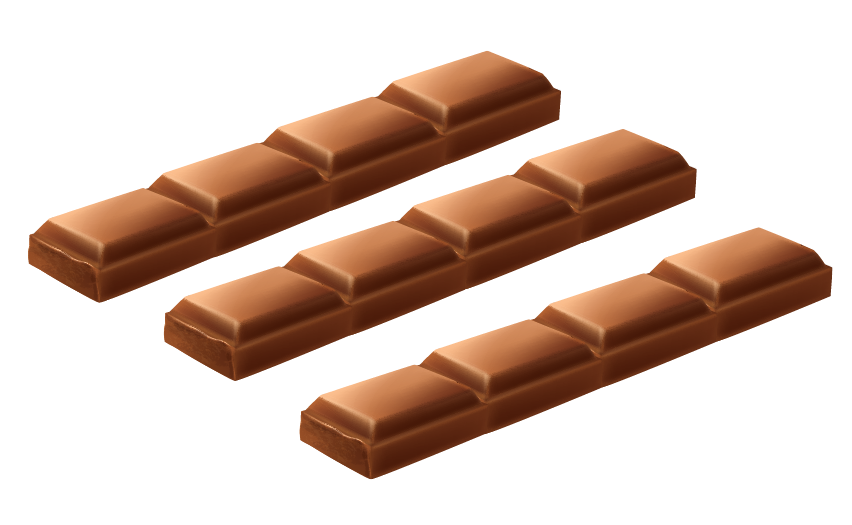The division statement for the picture above can be written as

$12 \div 3=4$

Imagine you have a chocolate bar with 12 pieces. You want to share it with your friend.

Can the bar be divided equally between the two? Will there be any leftover pieces?As you can see here, we have divided this bar of chocolate into 2 parts.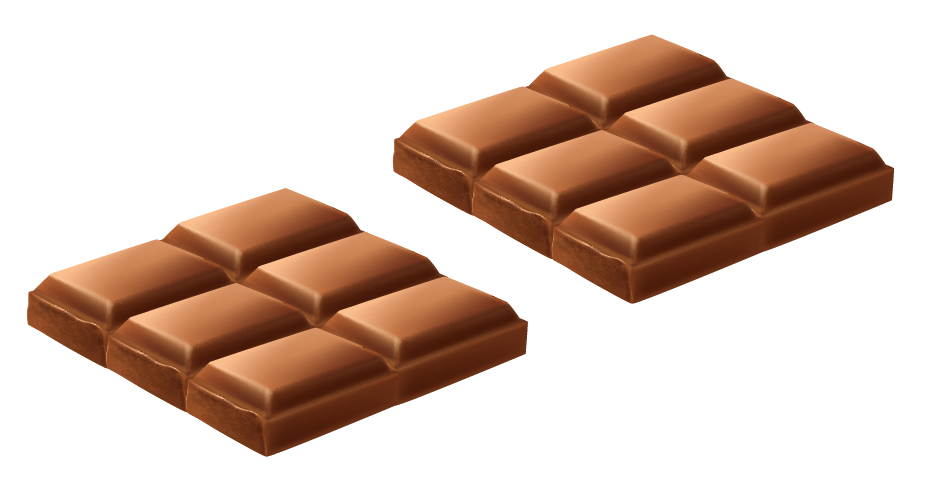Both you and your friend will get 6 pieces of chocolate each.

Did you notice? No piece of chocolate remains unshared. Hence, there is no remainder.

We can write the division statement for the example above as

$12 \div 2=6$

The above example can be summarised in the table below:

Terms Descriptions Values
Dividend The total pieces that are to be shared $$12$$
Divisor The number of equal groups that are to be made $$2$$
Quotient The number of pieces in each group $$6$$
Remainder The remaining piece that is not part of any group $$0$$

This example can also be mathematically represented as below: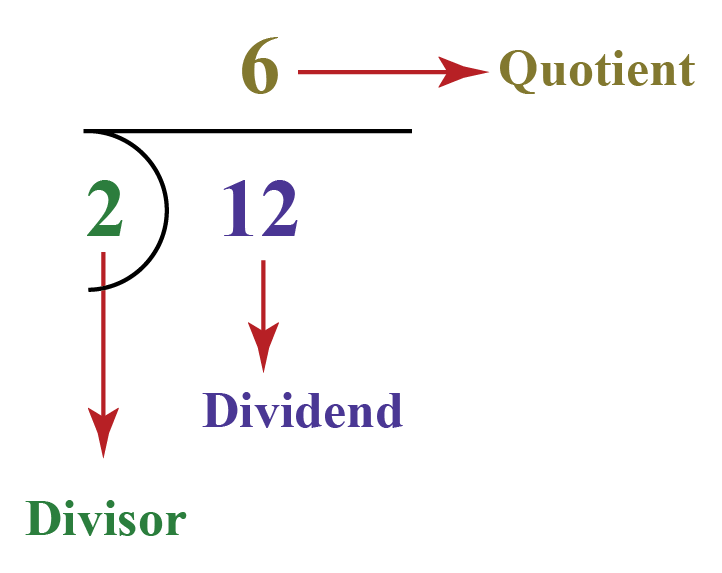## What are Quotients?

Quotient in mathematics can be defined as the result of the division between a number and any divisor.

It is the number of times the divisor is contained in the dividend without the remainder being negative.

In the image given below, the divisor $$2$$ is contained $$6$$ times in the dividend $$12$$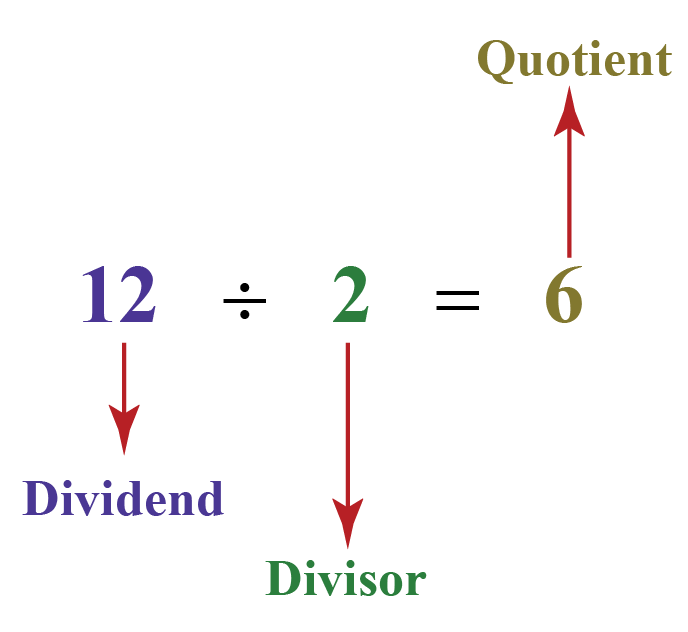## Long Division Method

This is the most common method used to solve problems on division.

Let us understand this with the help of examples.

### Steps of Long Division method

Let us solve $$435 \div 4$$.

The number 435 can be represented on an abacus as shown below: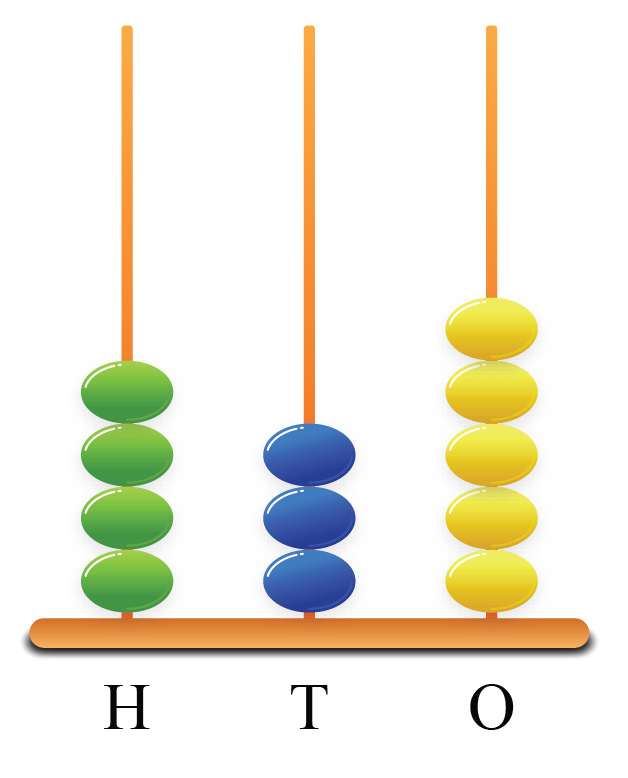Step 1:

Take the first digit of the dividend.

If this digit is greater than or equal to the divisor, then divide it by the divisor and write the answer on top.

Subtract the result from the digit and write below.

• Here, the first digit is 4 and it is equal to the divisor.
• So, $$4 \div 4 =1$$; 1 is written on top, inside the yellow box below.
• The result $$4 \times 1 =4$$ is subtracted from the digit and 0 is written below.
• Next drop the second digit or the digit in the ten’s place beside 0.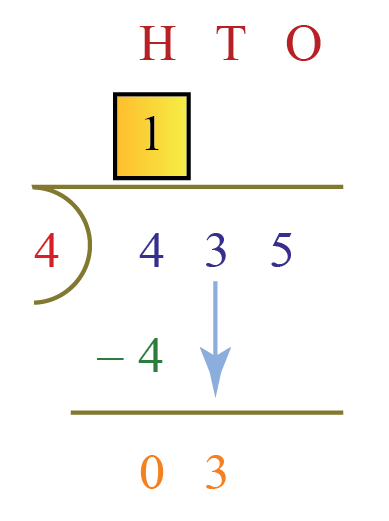Step 2:

We can see that we have 03 as the result of step 1.

• Repeat the same step of checking if this number is greater or smaller than the divisor.
• Since 03 is less than 4, we cannot divide this number.
• Hence, we write a 0 on the top and drop the digit on the unit place beside 3.

Now, we have 35.

• As 35 > 4, we can divide this number and write $$35 \div 4 = 8$$ on top.
• Subtract the result $$4 \times 8 = 32$$ from 35 and write 3.

3 is known as remainder and 108 is called the quotient.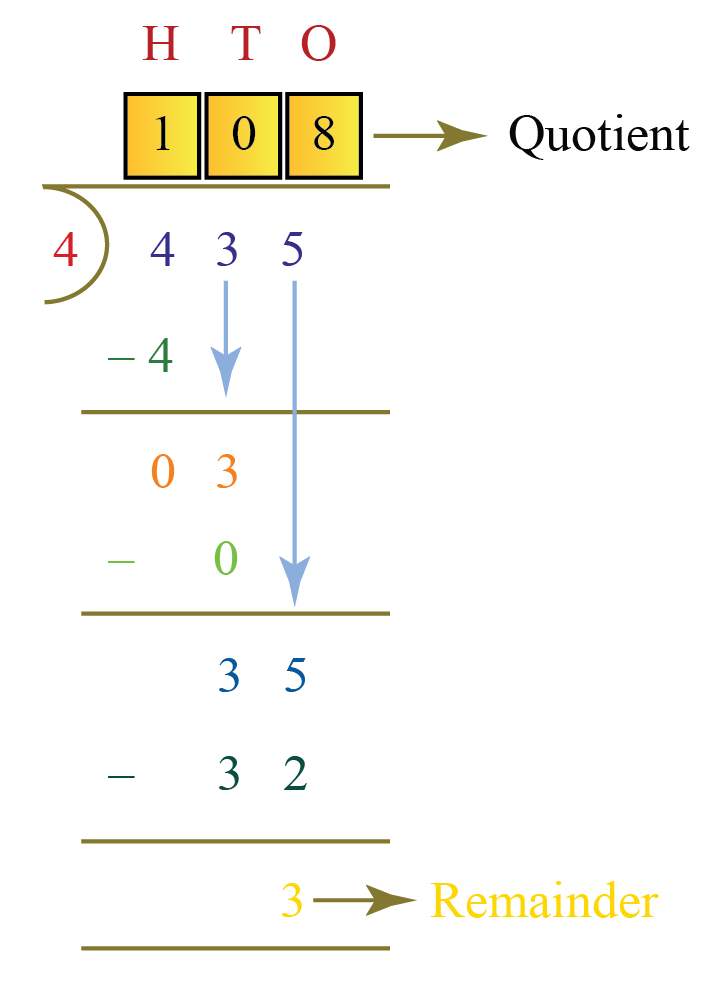Consider another example $$735 \div 9$$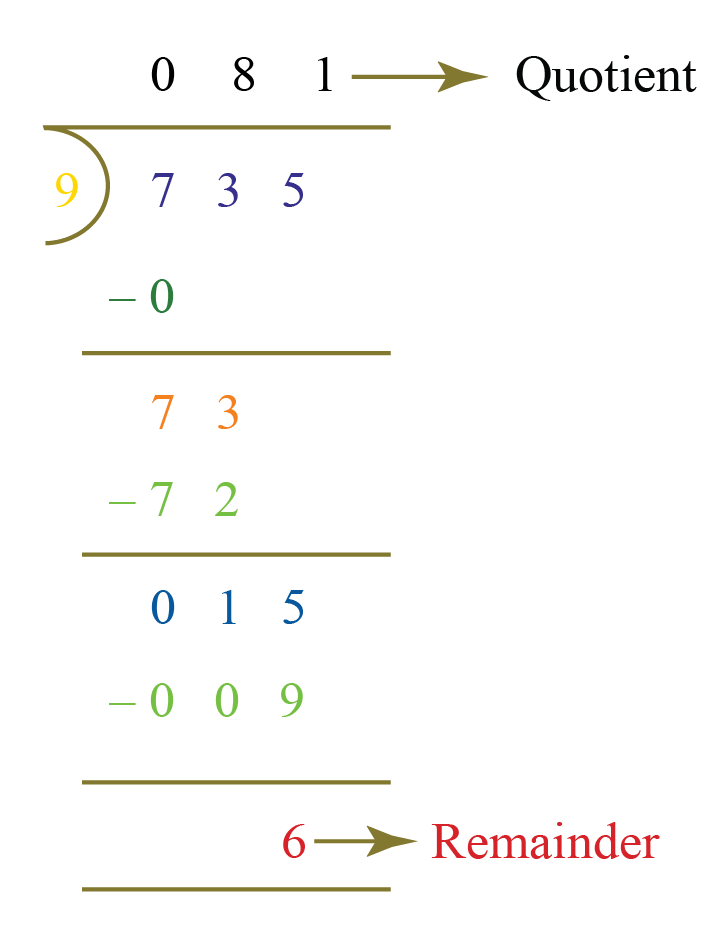In the above example, we can see that first digit of the dividend is less than the divisor.

Hence, we add a zero and drop the next digit to proceed with the calculation.

We consider the first 2 digits to proceed with the division.

Here, the quotient is 81 and the remainder is 6.

### Verification of Division Result

We can easily verify if our answer is correct or wrong.

As division is the reverse of multiplication, let us find out how we can verify our answer using this information.

For example,

$6 \div 2 = 3, \text { remainder} = 0$

In other words,

$6 = 2 \times 3 + 0$

This can be expressed as:

 Dividend = (Divisor $$\times$$ Quotient) $$+$$ Remainder

Let us reconsider the example disc

Let us reconsider the example discussed above, where the

• dividend = $$435$$
• divisor = $$4$$
• quotient = $$108$$
• remainder = $$3$$

Substituting the value in the formula, we get

$435 = \left(4 \times 108 \right) + 3$Tips and Tricks
1. Division is repeated subtraction.
So we can check our quotient by repeated subtraction as well.
2. We can check the quotient and the remainder of division using the following formula:
Dividend = (Divisor $$\times$$ Quotient) + Remainder
3. If the remainder is $$0$$, then we can check our quotient by multiplying it with the divisor.
If the product is equal to the dividend, then the quotient is correct.

## Quotient Calculator

The following "Quotient Calculator" is used to find the quotient when a bigger number is divided by another smaller number.

## Solved Examples

 Example 1

Rs. $$4000$$ is distributed among $$25$$ women for the work completed by them at a construction site. Calculate the amount given to each woman.

Solution:

The total amount = Rs. $$4000$$

The number of women at work = $$25$$

We have to calculate the amount given to each woman.

To do so, we have to divide $$4000$$ by $$25$$ using the long division method.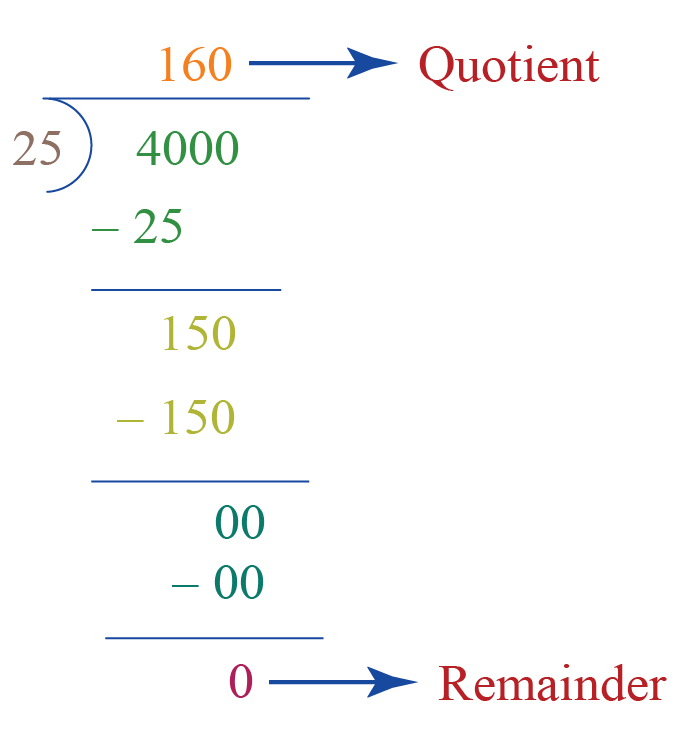Each woman will be given Rs. $$160$$

 $$\therefore$$ Amount given = Rs. $$160$$
 Example 2

$$66$$ people are invited to a birthday party. The suppliers have to arrange tables for the invitees. $$7$$ people can sit around a table.

How many tables should the suppliers arrange for the invitees?

Solution:

Total number of invitees = $$66$$

The number of people who can sit around one table = $$7$$

We divide $$66$$ by $$7$$ using the long division method.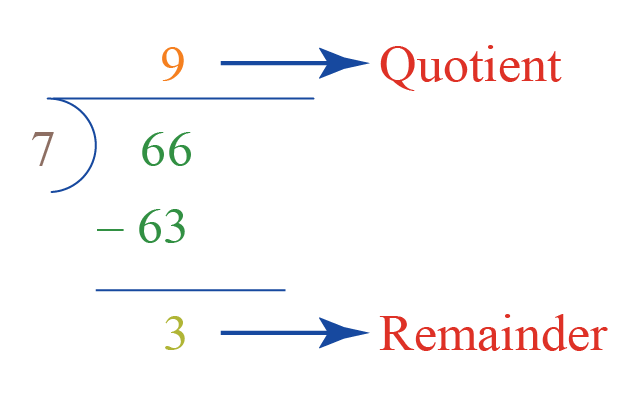Since $$9$$ is the quotient, we need $$9$$ tables.

With 9 tables, we will still have $$3$$ (which is the remainder) people without a place to sit.

Hence, the suppliers should arrange one more table for them.

The total number of tables to be arranged by the suppliers = $$9+1=10$$

 $$\therefore$$ Required number of tables = $$10$$
 Example 3

Calculate the number of hours in $$2100$$ minutes.

Solution:

We know that,

$$1$$ hour = $$60$$ minutes.

To find the number of hours in $$2100$$ minutes, we have to divide $$2100$$ by $$60$$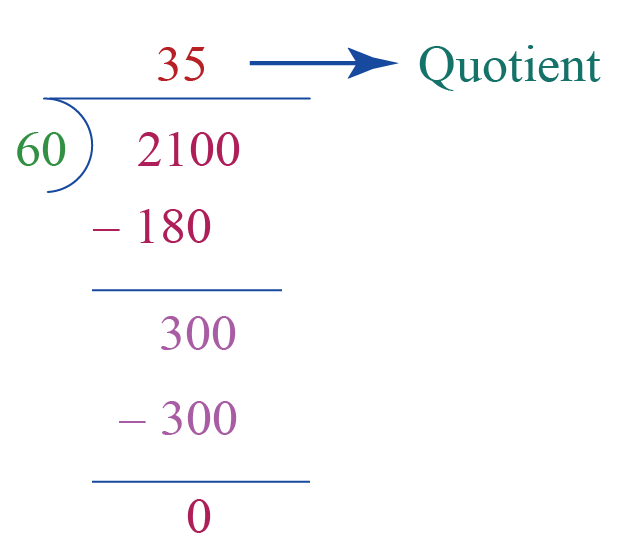There are $$35$$ hours in $$2100$$ minutes.

 $$\therefore$$ Required number of hours = $$35$$
 Example 4

When the teacher of class 6 asked a question, some students raised their hands. But instead of raising one hand, each student raised both their hands.

If there are $$56$$ hands in total, how many students raised their hands?

Solution:

The number of hands raised = $$56$$

To find the number of students who raised their hands, we have to divide $$56$$ by $$2$$ because each student raised both hands.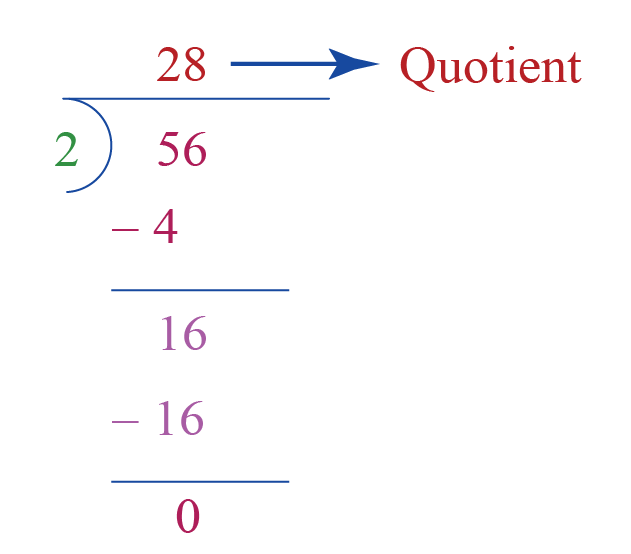Therefore, $$28$$ students raised their hands.

 $$\therefore$$ The number of students = $$28$$
 Example 5

Emma needs $$3$$ apples to make a big glass of apple juice. If she has $$51$$ apples, how many glasses of juice can she make?

Solution:

The total number of apples with Emma = $$51$$

The number of apples needed for one glass of juice = $$3$$

To find the number of glasses of juice, we divide $$51$$ by $$3$$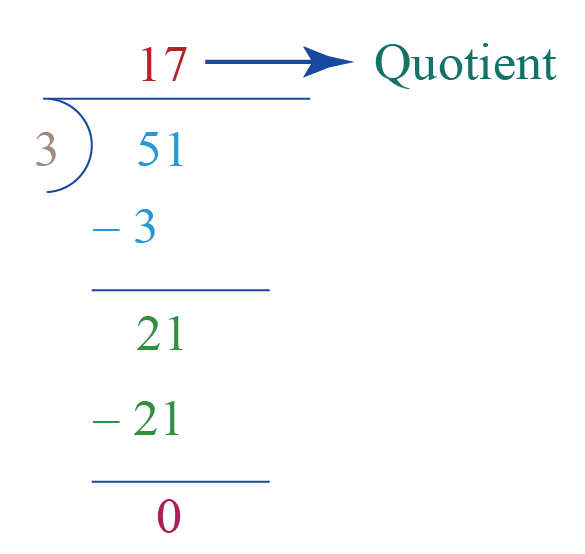Emma can make $$17$$ glasses of juice with 51 apples.

 $$\therefore$$ Number of glasses of juice = $$17$$

## Practice QuestionsChallenging Questions
1. Find the quotient when $$3242$$ is divided by $$13$$
2. Joseph has $$52$$ cards. He arranges them into $$4$$ groups. How many cards do each group contain?
3. Mary distributed $$32$$ scoops of ice cream into $$8$$ cones evenly. How many scoops of ice cream does each cone contain?
4. There are $$100$$ toes in a swimming pool.  If each person has $$10$$ toes, how many people are there in the swimming pool?

IMO (International Maths Olympiad) is a competitive exam in Mathematics conducted annually for school students. It encourages children to develop their math solving skills from a competition perspective.

## 1. What is division?

Division is the process of splitting a larger group into equal groups.

## 2. What is dividend and divisor?

The larger group which is to be split is known as the dividend.

Divisor is the number of units required in each smaller group.

If the question is $$63 \div 9$$, then 63 is the dividend and 9 is the divisor.

## 3. What is quotient in maths (definition)?

The result of division is known as quotient in mathematics.

In the example $$63 \div 9$$, 7 will be the quotient since 7 groups can be formed with 9 units in each group.

## 4. What is remainder?

The number of remaining units which cannot be part of any smaller group is known as the remainder.

## 5. How can we verify the answer in division?

Division is also known as inverse multiplication.

We can verify our results by using the following formula: Dividend = (Divisor x Quotient) + Remainder

## 6. How do you find the quotient in math?

We can find the quotient using the long division method. You can refer to the "Long Division Method" section of this page for examples.

## 7. What is the quotient of 63 and 9?

We know that $$9 \times 7 = 63$$

Therefore, if we we divide $$63$$ by $$9$$, the quotient is $$7$$

## 8. What is the quotient of $$7$$ and $$2$$?

We know that $$2 \times 3 =6$$ and $$2 \times 4 =8$$

Therefore, the quotient when $$7$$ divided by $$2$$ is $$3$$ (because the next product $$8$$ is more than $$7$$)

More Important Topics
Numbers
Algebra
Geometry
Measurement
Money
Data
Trigonometry
Calculus